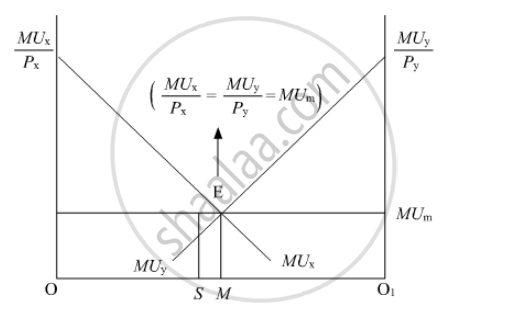Advertisement Remove all ads

# “A consumer consumes only two goods X and Y”. State and explain the conditions of consumer’s equilibrium with the help of utility analysis. - Economics

Advertisement Remove all ads
Advertisement Remove all ads
Advertisement Remove all ads

“A consumer consumes only two goods X and Y”. State and explain the conditions of consumer’s equilibrium with the help of utility analysis.

Advertisement Remove all ads

#### Solution

In case of two commodities, the consumer’s equilibrium is attained in accordance with the Law of Equi-Marginal Utility. It states that a consumer allocates his expenditure on two goods in such a manner that the utility derived from each additional unit of the rupee spent on each of the commodities is equal. That is,

Marginal Utility of a Rupee spent on commodity = Marginal Utility of a Rupee spent on commodity y = Marginal Utility of Money

Or           (Mu_x)/P_x=(MU_y)/(P_y)=Mu_mIn the diagram, OO1 represents the total income of a consumer. MUand MUrepresent the Marginal Utility curves of commodity and commodity Y, respectively. Equilibrium is established at point E, where, MUand MUintersect each other and with MUy.

At this point, OM amount of income is spent on commodity X and the remaining amount of income MO1 is spent on commodity Y.

Suppose, instead of point M, the consumer is at point S, where he spends OS amount of income on commodity X and SO1 amount of income on commodity Y. At point S, however;

(MU_x)/(P_x)>(MU_y)/(P_y)

Thus, the consumer would increase his consumption of commodity X till the equality is achieved. That is, in other words, the consumer increases his consumption of good till he reaches point where,

(MU_x)/(P_x)=(MU_y)/(P_y)

Concept: Marginal Rate of Substitution (MRS)
Is there an error in this question or solution?

#### APPEARS IN

Advertisement Remove all ads
Share
Notifications

View all notifications

Forgot password?